Note: The other languages of the website are Google-translated. Back to English

## Count lines which are separated by line breaks in a cell or a range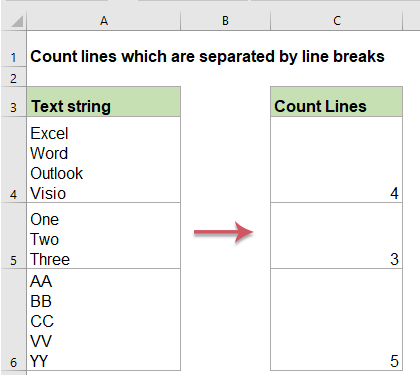This article is talking shout how to count the number of lines which are separated by line breaks in a cell or a range of cell by using formula in Excel.

Count the number of lines in a cell

Count the number of lines in a range of cells

#### Count the number of lines in a cell

To count total lines in a cell, you can use the combination of the LEN, SUBSTITUTE and CHAR functions.

1. Please apply the below formula:

=LEN(A2)-LEN(SUBSTITUTE(A2,CHAR(10),""))+1

2. And then, drag the fill handle down to the cells that you want to apply this formula, and all lines in each cell have been counted, see screenshot: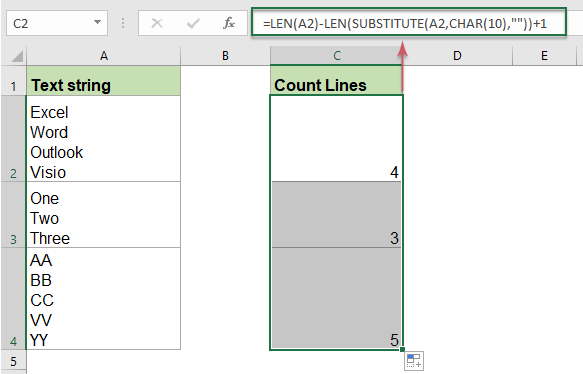##### Explanation of the formula:
• LEN(A2): count the number of total characters in cell A2;
• SUBSTITUTE(A2,CHAR(10),""): is used to replace all line break characters with empty characters in cell A2;
• LEN(SUBSTITUTE(A2,CHAR(10),"")): count the number of all the characters in cell A2 without line break characters;
• LEN(A2)-LEN(SUBSTITUTE(A2,CHAR(10),""))+1: the first count subtracts the second count, and add 1 to get the result.

Note: With the above formula, you will get the result 1 if a cell is empty, to overcome this problem, please apply the following formula:

=LEN(A2)-LEN(SUBSTITUTE(A2,CHAR(10),""))+(LEN(A2)>1)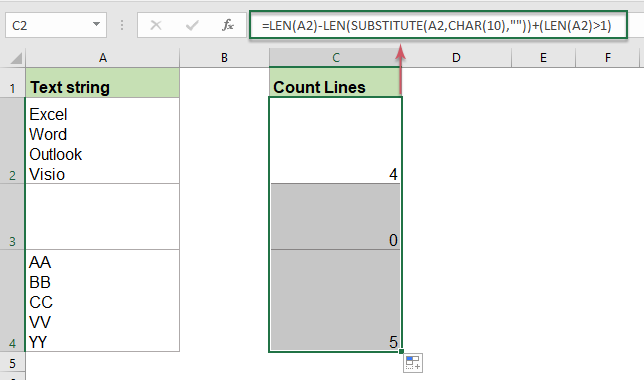#### Count the number of lines in a range of cells

If you need to count the total number of lines from a range of cells, the following formulas ca help you.

1. Enter or copy the below formula into a blank cell where you want to output the result:

=SUM(LEN(A2:A4)-LEN(SUBSTITUTE(A2:A4,CHAR(10), ""))+1)

2. And then, press Ctrl + Shift + Enter keys together, and the number of the total lines in a range has been calculated, see screenshot: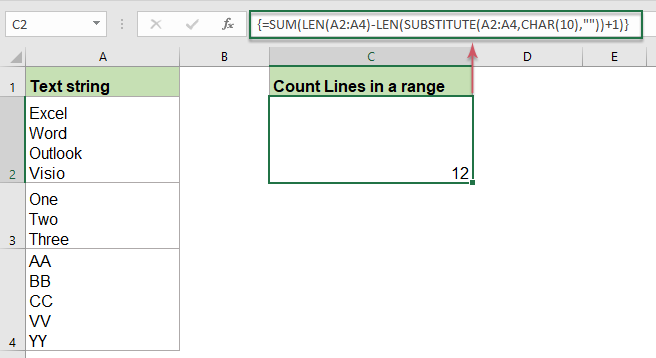Note: To fix the above formula when there are blank cells in the range, please use the below formula:

=SUMPRODUCT(LEN(A2:A4)-LEN(SUBSTITUTE(A2:A4,CHAR(10),""))+(LEN(A2:A4)>0))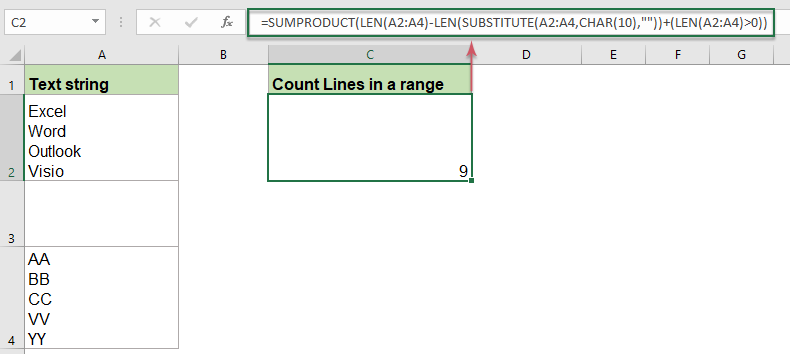#### Relative functions used:

• LEN:
• The LEN function returns the number of characters in a text string.
• SUBSTITUTE:
• The Excel SUBSTITUTE function replaces text or characters within a text string with another text or characters.

#### More articles:

• Count Keywords Cell Contains Based On A List
• If you want to count the number of keywords appears in a cell based on a list of cells, the combination of the SUMPRODUCT, ISNUMBER and SEARCH functions may help you to solve this problem in Excel.
• Show specific text based on value in Excel
• Supposing, you have a list of values, now, you would like to show a specific text based on the value. For example, if cell value is greater than 1000, then “Increase” text is displayed in another column as below screenshot shown. To deal with this task, both the REPT and IF functions can do you a favor.
• Check if cell equals any value in list
• For example, I have a list of text strings in column A, and now, I want to check if cell value in this column equals any value in another list of column E. If equals, display TRUE, otherwise, a FALSE value is displayed as below screenshot shown.

### The Best Office Productivity Tools

#### Kutools for Excel - Helps You To Stand Out From Crowd

Would you like to complete your daily work quickly and perfectly? Kutools for Excel brings 300 powerful advanced features (Combine workbooks, sum by color, split cell contents, convert date, and so on...) and save 80% time for you.

• Designed for 1500 work scenarios, helps you solve 80% Excel problems.
• Reduce thousands of keyboard and mouse clicks every day, relieve your tired eyes and hands.
• Become an Excel expert in 3 minutes. No longer need to remember any painful formulas and VBA codes.
• 30-day unlimited free trial. 60-day money back guarantee. Free upgrade and support for 2 years.#### Office Tab - Enable Tabbed Reading and Editing in Microsoft Office (include Excel)

• One second to switch between dozens of open documents!
• Reduce hundreds of mouse clicks for you every day, say goodbye to mouse hand.
• Increases your productivity by 50% when viewing and editing multiple documents.
• Brings Efficient Tabs to Office (include Excel), Just Like Chrome, Firefox, And New Internet Explorer.